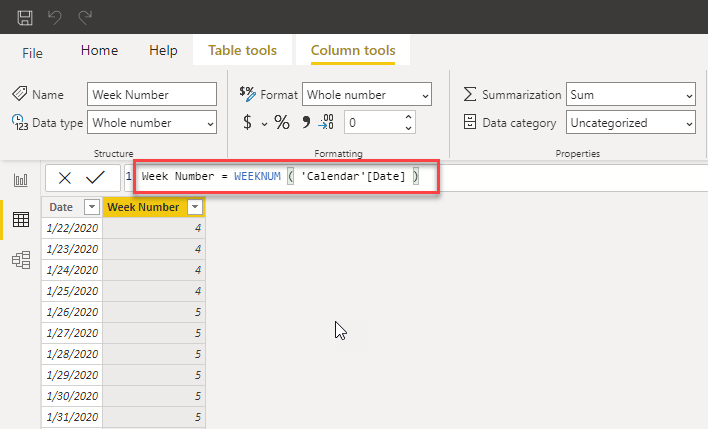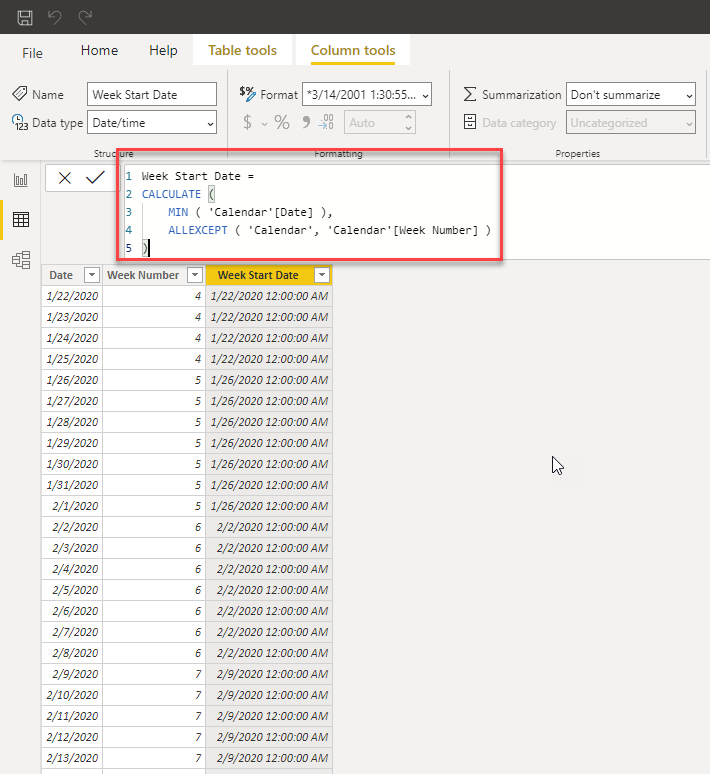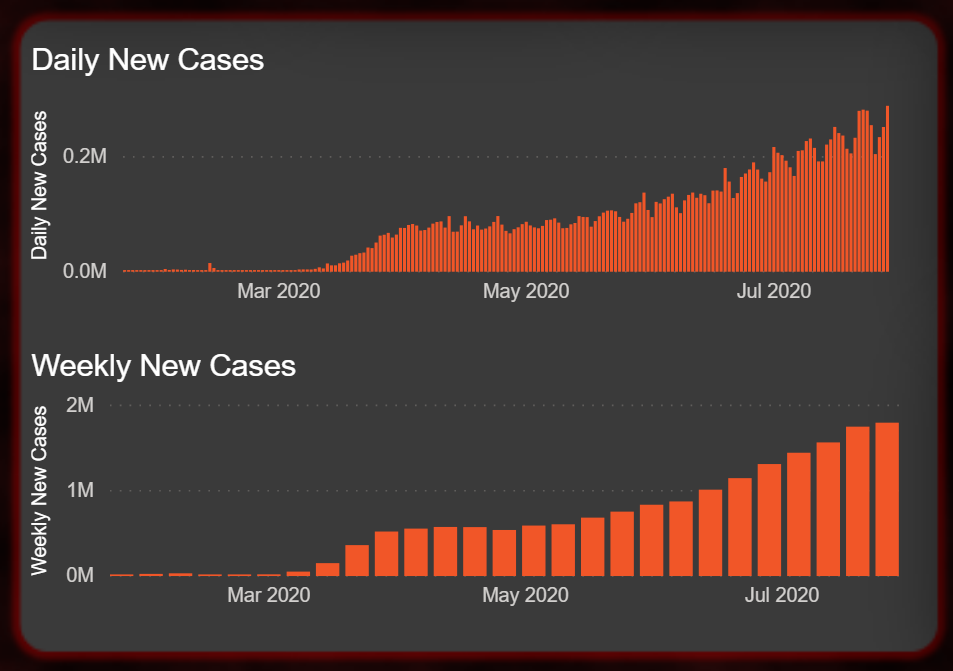Search
• Reviewed by Maslows

# Weekly-Based Time Intelligence in Power BI from DataChant

In one of my last tutorials here, we learned how to apply time-intelligence in DAX to analyze the COVID-19 data that is provided by Johns Hopkins University. The focus so far was to provide correct calculations on a daily level or to smooth them using running averages here.

In today’s quick tutorial, you will learn how to add weekly-based calculations. You can download the pbix file from here to use as the base-line for this tutorial.

We will start by creating a calculated column in your Calendar table for the week number. Click on the Data view (The second icon on the left sidebar). In the Fields pane on the right sidebar, select the Calendar table and click New column under Table tools.Enter the following expression in the formula bar:

`Week Number = WEEKNUM ( 'Calendar'[Date] )`

This WEEKNUM function will return the week number (for more options to set the week number go here). For example, for the date of January 22, 2020, the week number will be 4 since that date is in the fourth week in 2020.Now you can use this column in the X-axis of your visualizations and apply your measures.

### When WEEKNUM is not enough

When you use the Week Number in the X-axis as illustrated in the top bar chart below, your audience may find it a bit difficult to translate a week number with the actual date. In this section, you will learn how to translate the week number to the first date of the week and use it as illustrated in the bottom bar chart below.When Week Number is not enough. How do conver week numbers to dates of the first day of the week

Go to Table tools and click Calculated column. In the formula bar, enter the formula below.

`Year = YEAR ( 'Calendar'[Date] )`

Note: You may already have a Year column in your calendar table. It can help you if you build your own date hierarchy in Power BI (You would also need to include the Month column). But in this tutorial, we need the Year column to ensure that we calculate the week start date correctly.

Add another calculated column with this formula:

```Week Start Date =
CALCULATE (
MIN ( 'Calendar'[Date] ),
ALLEXCEPT ( 'Calendar', 'Calendar'[Week Number], 'Calendar'[Year] )
)```If you need to apply weeks’ end dates in your analysis, here is your modified DAX formula (We only changed the MIN to MAX):

```Week End Date =
CALCULATE (
MAX ( 'Calendar'[Date] ),
ALLEXCEPT ( 'Calendar', 'Calendar'[Week Number], 'Calendar'[Year] )
)```

Let’s explain the code fo the calculated column of Week Start Date. In its core, we apply a MIN on the Date column to get the earliest date that we have for all the records with the same Week Number and Year. To get to the necessary calculation you would need to modify the filter context in DAX to support the requirement in bold above.

Without CALCULATE, the formula MIN( ‘Calendar'[Date] ) will return the earliest date in the entire calendar table, because there is no filter context when you run calculated columns.

The formula CALCULATE ( MIN( ‘Calendar'[Date] ) ) will return the same date you have in each row since the row context is converted into a filter context that includes the selected date.

To modify the filter context in CALCULATE and get all the rows with the same week number and year, we apply the ALLEXCEPT function that will return all the raws in the Calendar table except Week Number and Year which will not be filtered. As a result, rows with different Week Number or Year values will not be included in the result. This operation will return to us the affected result. The MIN function will run only on all the rows in the table with the same Week Number and Year and return the first date of the week.

Note: ALLEXCEPT is a less common function in DAX than ALL. As a result, you may already know how to achieve the same Week Start Date by applying ALL as shown in the formula below, but the function below is not efficient as it will iterate over all the days in the Calendar table.

```Week Start Date (Don't use it) =
VAR _weekNum = 'Calendar'[Week Number]
VAR _year = 'Calendar'[Year]
RETURN
CALCULATE (
MIN ( 'Calendar'[Date] ),
FILTER (
ALL ( 'Calendar' ),
'Calendar'[Week Number] = _weekNum &&
'Calendar'[Year] = _year
)
)```

Now you have the Week Start Date as a calculated column. It’s time to format it as a date.Now, when we have the Week Start Date column we can place it in the X-axis of our visuals and analyze our data by weeks. In one of my previous tutorials here on building the COVID-19 dashboard, we used Time Intelligence to calculate the daily new cases. Here is the equivalent measure that calculates the weekly new cases:

```Weekly New Cases =
VAR current_day = [Total Confirmed]
VAR prev_day =
CALCULATE (
[Total Confirmed],
DATEADD ( 'Calendar'[Date], -7, DAY )
)
RETURN
IF ( ISBLANK ( prev_day ), BLANK(), current_day - prev_day )```

By applying the Weekly New Cases in conjunction with the Week Start Date column you can now analyze the weekly new cases of confirmed cases of COVID-19 by weeks and use the week dates instead of numbers.I hope you found this tutorial useful. In my next blog post, we will continue our exploration of PDF import as started here.

The post Weekly-Based Time Intelligence in Power BI appeared first on DataChant.